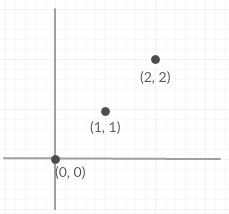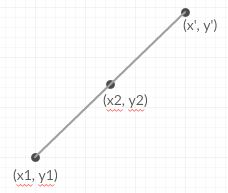Reflection of a point at 180 degree rotation of another point

• Last Updated : 26 Feb, 2021

Given two points coordinates (x1, y1) and (x2, y2)on 2D plane. The task is to find the reflection of (x1, y1) at 180 degree rotation of (x2, y2).
Examples:

Input : x1 = 0, y1 = 0, x2 = 1, y2 = 1
Output : (2, 2)Input : x1 = 1, y1 = 1, x2 = 2, y2 = 2
Output : (3, 3)

Let the reflection point of point (x1, y1) about (x2, y2) be (x’, y’).
For (x’, y’) be the 180 degree rotation of point (x1, y1) around point (x2, y2), they all must be collinear i.e all the three point must lie on a same straight line. Also, observe (x2, y2) will became mid point between (x1, y1) and (x’, y’).So,
x’ – x2 = x2 – x1
y’ – y2 = y2 – y1
x’ = 2 * x2 – x1
y’ = 2 * y2 – y1
Below is the implementation of this approach:

C++

 // CPP Program tof find the 180 degree reflection// of one point around another point.#include using namespace std; void findPoint(int x1, int y1, int x2, int y2){    cout << "(" << 2 * x2 - x1 << ", "         << 2 * y2 - y1 << ")";}  int main(){    int x1 = 0, y1 = 0, x2 = 1, y2 = 1;    findPoint(x1, y1, x2, y2);    return 0;}

Java

 // Java Program to find the 180 degree// reflection of one point around// another point.class GFG {         static void findPoint(int x1, int y1,                          int x2, int y2)    {        System.out.println("(" + (int)(2 * x2 - x1)               + "," + (int)(2 * y2 - y1 ) + " )");    }         // Driver code    public static void main(String args[])    {        int x1 = 0, y1 = 0, x2 = 1, y2 = 1;                 findPoint(x1, y1, x2, y2);    }} // This code is contributed by Arnab Kundu.

Python3

 # Python3 Program for find the 180# degree reflection of one point# around another point. def findPoint(x1, y1, x2, y2):    print("(" , 2 * x2 - x1 , ",",                2 * y2 - y1 ,")"); # Driver Codex1 = 0;y1 = 0;x2 = 1;y2 = 1;findPoint(x1, y1, x2, y2); # This code is contributed by mits

C#

 // C# Program to find the 180 degree reflection// of one point around another point.using System; public class GFG {         static void findPoint(int x1, int y1,                          int x2, int y2)    {        Console.WriteLine("(" + (int)(2 * x2 - x1)               + "," + (int)(2 * y2 - y1 ) + " )");    }         // Driver code    static public void Main(String []args)    {        int x1 = 0, y1 = 0, x2 = 1, y2 = 1;                 findPoint(x1, y1, x2, y2);    }} // This code is contributed by Arnab Kundu.



Javascript


Output:
(2, 2)

Time Complexity : O(1)

My Personal Notes arrow_drop_up